# Class 12 Maths NCERT Solutions for Chapter 7 Integrals Exercise 7.9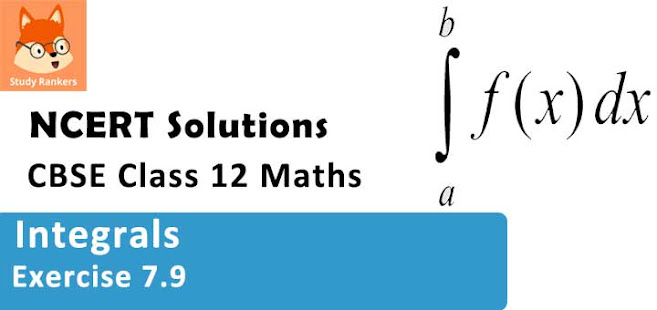### Integrals Exercise 7.9 Solutions

1. Evaluate the definite integrals -11­ (x + 1) dx

Solution

Let I =  -11­ (x + 1) dx
∫(x + 1)dx = x2­/2 + x = F(x)
By second fundamental theorem of calculus, we obtain
I = F(1) - F(-1)
= (1/2 + 1) - (1/2 - 1)
= (1/2 + 1 - 1/2 + 1)
= 2

2. Evaluate the definite integrals  23­ (1/x) dx

Solution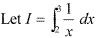∫(1/x) dx = log |x| = F(x)
By second fundamental theorem of calculus, we obtain
I  = F(3) - F(2)
= log|3| - log|2| = log (3/2)

3. Evaluate the integrals in using substitution 01­ sin-1­ [2x/(1 + x2­ ) dx
Solution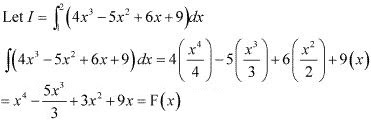By second fundamental theorem of calculus, we obtain
I = F(2) - F(1)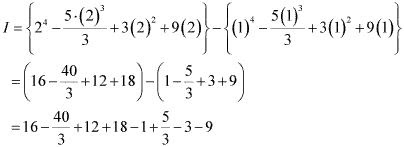= 33 - (35/3)
= (99 - 35)/3
= 64/3

4. Evaluate the definite integrals 0x/4­ sin2x dx
Solution
Let I = 0x/4­ sin2x dx
sin 2x dx = (- cos 2x/2) = F(x)
By second fundamental theorem of calculus, we obtain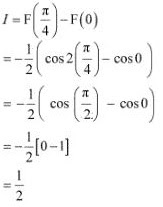5. Evaluate the definite integrals 0x/2­ cos 2x dx
Solution
Let I =  0x/2­ cos 2x dx
∫cos 2x dx = (sin 2x/2) = F(x)
By second fundamental theorem of calculus, we obtain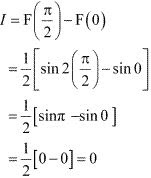6. Evaluate the definite integrals 45­ ex­ dx
Solution
Let I =  45­ ex­ dx
∫ex­ dx = ex­ = f(x)
By second fundamental theorem of calculus, we obtain
I = F(5) - F(4)
= e5­ - e4­
= e4­ (e - 1)

7. Evaluate the definite integrals 0x/4­ tan x dx
Solution
Let I = ­ 0π/4­ tan x dx
∫tan x dx = -log |cos x| = F(x)
By second fundamental theorem of calculus, we obtain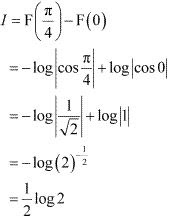8. Evaluate the definite integrals ∫π/6 π/4 cosec x dx
Solution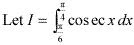∫(cosec x) dx = log | cosec x - cot x| = F(x)
By second fundamental theorem of calculus, we obtain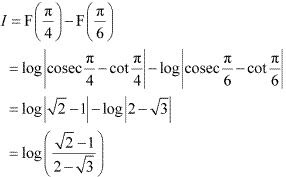9. Evaluate the definite integrals 04 dx/(√ 1 - x2­ )
Solution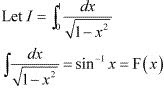By second fundamental theorem of calculus, we obtain
I = F(I) - F(0)
= sin-1­ (1) -  sin-1­ (0)
= (π/2) - 0
= π/2

10. Evaluate the definite integrals 04  dx/(1 + x2­ )
Solution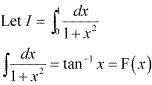By second fundamental theorem of calculus, we obtain
I = F(1) - F(0)
= tan-1­ (1) - tan-1­ (0)
= π/4

11. Evaluate the definite integrals 23 dx/(x2­ - 1)
Solution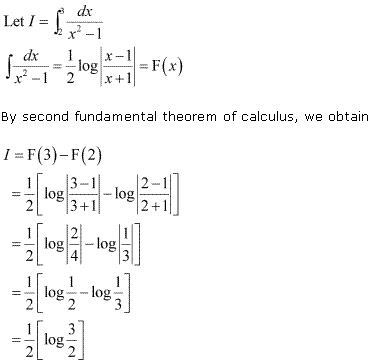12. Evaluate the definite integrals 0π/4­ cos2­x dx
Solution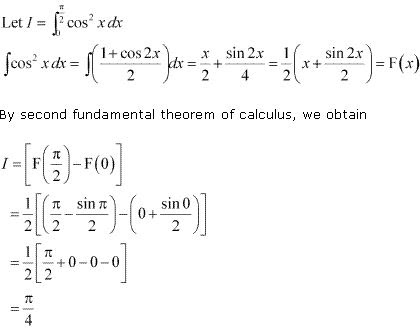13. Evaluate the definite integrals 23 xdx/(x2­ + 1)
Solution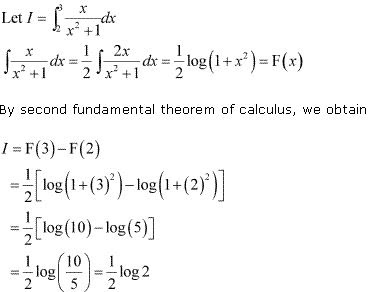14. Evaluate the definite integrals 01 (2x + 3)/(5x2­ + 1) dx
Solution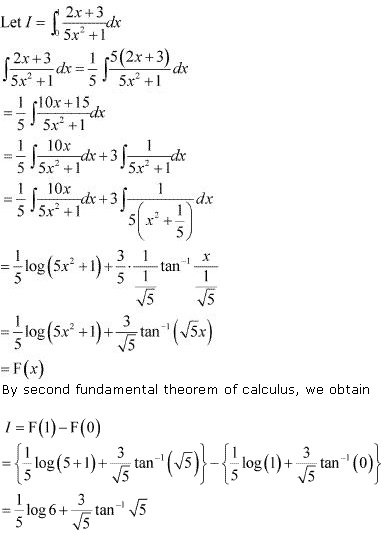15. Evaluate the definite integrals  01 xex2 dx
Solution
Let I = 01 xex2 dx
Put x2­ = t
⇒ 2x dx = dt
As x → 0, t → 0 and as x → 1, t →1,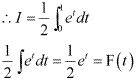By second fundamental theorem of calculus, we obtain
I = F(1) - F(0)
= (1/2)e - (1/2)e0­
= (1/2) (e - 1)

16. Evaluate the definite integrals  12 5x2­ /(x2­ + 4x + 3)
Solution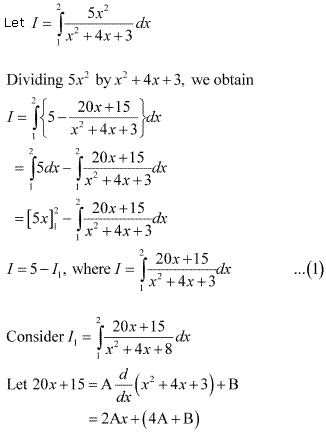Equating the coefficients of x and constant term, we obtain
A = 10 and B = -25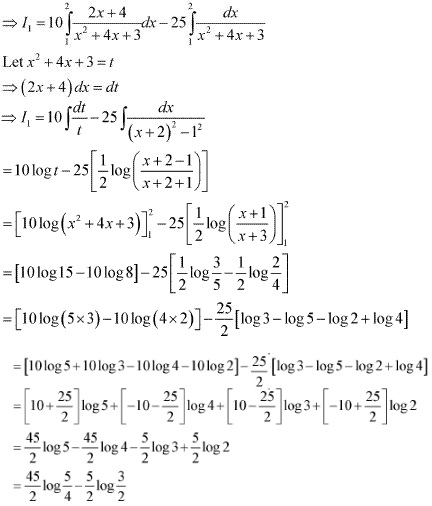Substituting the value of I1 in (1) , we obtain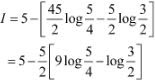17. Evaluate the definite integrals 0π/4­ (2 sec2­ x + x3­ + 2)dx
Solution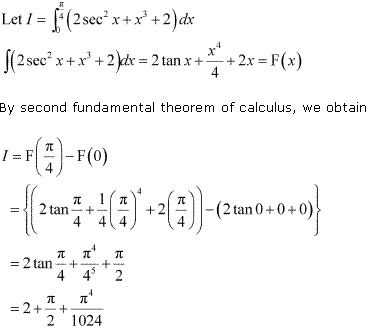18. Evaluate the definite integrals 0π­ (sin2­ x/2 - cos2­ x/2) dx
Solution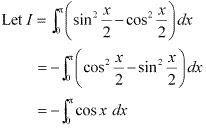∫(cos x) dx = sin x = F(x)
By second fundamental theorem of calculus, we obtain
I = F(π) - F(0)
= sinπ - sin 0
= 0

19. Evaluate the definite integrals  02 [(6x + 3)/(x2­ + 4)] dx
Solution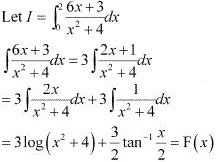By second fundamental theorem of calculus, we obtain
I = F(2) - F(0)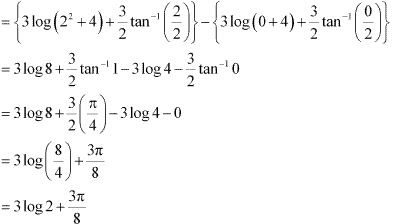20. Evaluate the definite integrals  01 [xex­ + sin(πx/4)]
Solution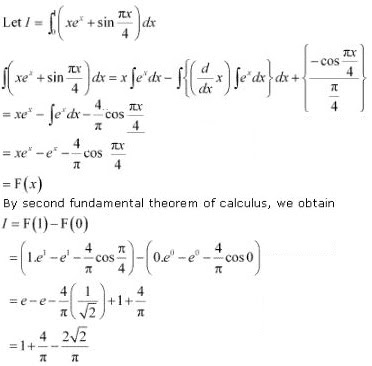21. Choose the correct answer  1√3 dx/(1 + x2­ ) equals
(A) π/3
(B) 2π/3
(C) π/6
(D) π/12
Solution
∫dx/(1 + x2­ ) = tan-1­ x = F(x)
By second fundamental theorem of calculus, we obtain= tan-1­ √3 - tan-1­ 1
= π/3 - π/4
= π/12
Hence, the correct answer is D.

22. Choose the correct answer 02/3­ dx/(4 + 9x2­ ) equals is
Solution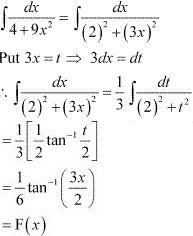By second fundamental theorem of calculus, we obtain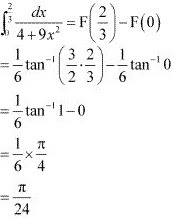Hence, the correct answer is C.